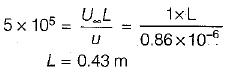Courses

# Test: Viscous Flow - 3

## 10 Questions MCQ Test Topicwise Question Bank for Mechanical Engineering | Test: Viscous Flow - 3

Description
This mock test of Test: Viscous Flow - 3 for Mechanical Engineering helps you for every Mechanical Engineering entrance exam. This contains 10 Multiple Choice Questions for Mechanical Engineering Test: Viscous Flow - 3 (mcq) to study with solutions a complete question bank. The solved questions answers in this Test: Viscous Flow - 3 quiz give you a good mix of easy questions and tough questions. Mechanical Engineering students definitely take this Test: Viscous Flow - 3 exercise for a better result in the exam. You can find other Test: Viscous Flow - 3 extra questions, long questions & short questions for Mechanical Engineering on EduRev as well by searching above.
QUESTION: 1

Solution:
QUESTION: 2

Solution:
QUESTION: 3

### For laminar flow is pipe, the kinetic energy correction factor is

Solution:
QUESTION: 4

Which one of the following is correct for the velocity distribution across a section of two fixed parallel plates having viscous flow, where t is the distance of parallel plate

Solution:
QUESTION: 5

What would be the shear stress distribution across a section of two fixed parallel plates kept at a distance t apart and having a viscous flow

Solution:
QUESTION: 6

An incompressible fluid (kinematic viscosity, 7.4 x 10-7 m2/s, specific gravity, 0.88) is held between two parallel plates. If the top plate is moved with a velocity of 0.5 m/s while the bottom one is held stationary, the fluid attains a linear velocity profile in the gap of 0.5 mm between these plates; the shear stress in Pascals on the surface of top plate is

Solution:

Shear stress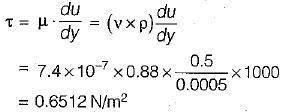QUESTION: 7

The maximum velocity of a one-dimensional incompressible fully developed viscous flow, between two fixed parallel plates, is 6 ms-1. The mean velocity (in ms-1) of the flow is

Solution:

Ratio of maximum velocity to average velocity for one dimensional incompressible flow between two fixed plate is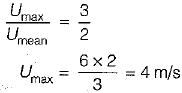QUESTION: 8

The shear stress developed in a lubricant oil viscosity 9.81 poise, filled between two parallel plates 1 cm apart and moving with relative velocity of 2 m/sec is

Solution: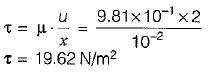QUESTION: 9

The drag coefficient for laminar flow varies with reynolds number (Re) as

Solution: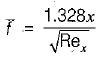QUESTION: 10

The transition Reynolds number for flow over a flat plate is 5 x 105. What is the distance from the leading edge at which transition will occur for flow of water with a uniform velocity of 1 m/s (for water the kinematic viscosity v = 0.86 x 10-6m2/s)

Solution: